# seaborn库各个画图函数使用实例

## 前言：

### 链接：https://pan.baidu.com/s/1ggSmjI7aOB7CqbDmVfvw0A 提取码：x4kv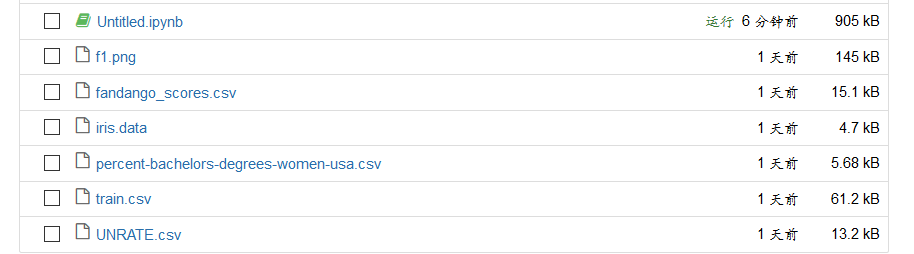## 1、seaborn的出现

x = np.random.normal(size=100)
sns.distplot(x,kde=False)

seaborn是matlibplot.pyplot的封装。seaborn有各种画图函数，其丰富的生态系统以及良好的画面感，使得seaborn越来越受用户们的青睐。

## 2、seaborn的使用

import seaborn as sns

## 3、seaborn函数实例（有图片）

### 1）、使用柱形图

x = np.random.normal(size=100)
sns.distplot(x,kde=False)

Out：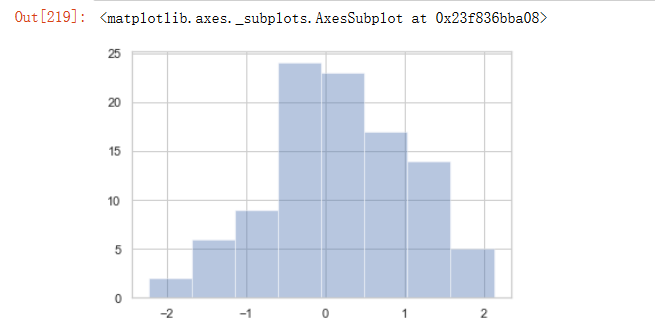### 2、使用点图

mean, cov = [0, 1], [(1, .5), (.5, 1)]
data = np.random.multivariate_normal(mean, cov, 200)
df = pd.DataFrame(data, columns=["x", "y"])
# df
sns.jointplot(x="x", y="y", data=df);

Out：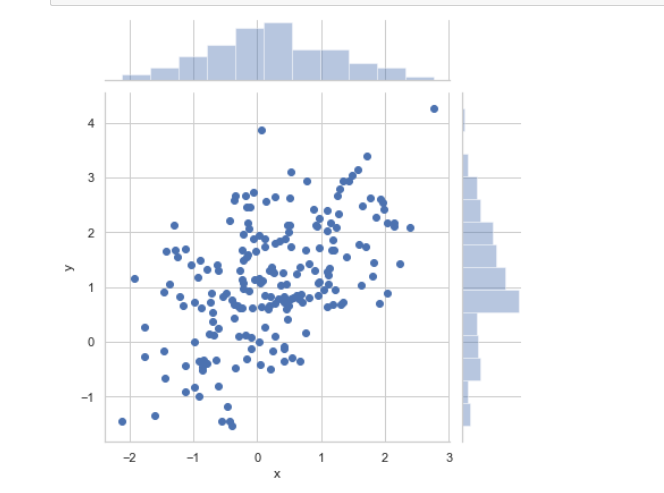### tip:当点过多，使用下面这个进行数据可视化

x, y = np.random.multivariate_normal(mean, cov, 1000).T
with sns.axes_style("white"):
sns.jointplot(x=x, y=y, kind="hex", color="black")

### 3、进行多变量分析

iris = sns.load_dataset("iris")
sns.pairplot(iris)
print (iris)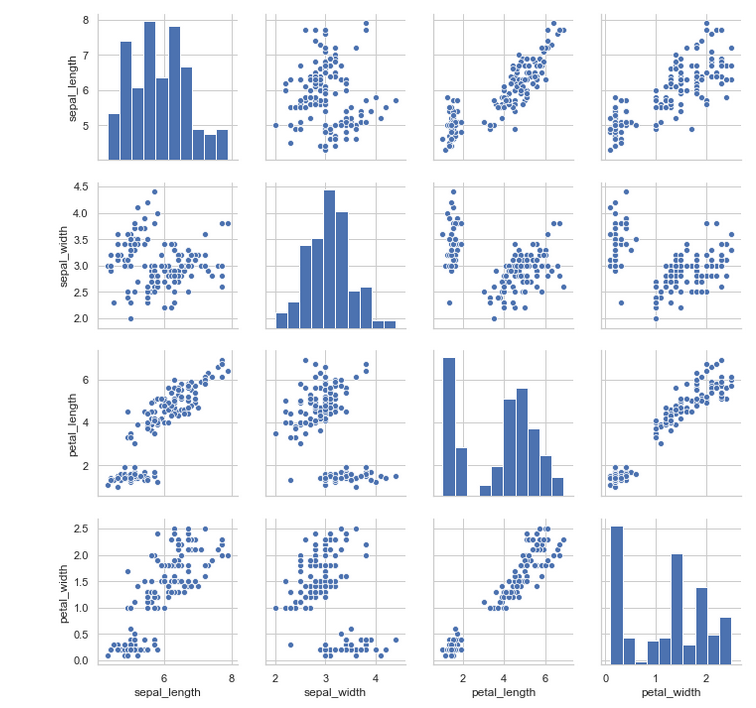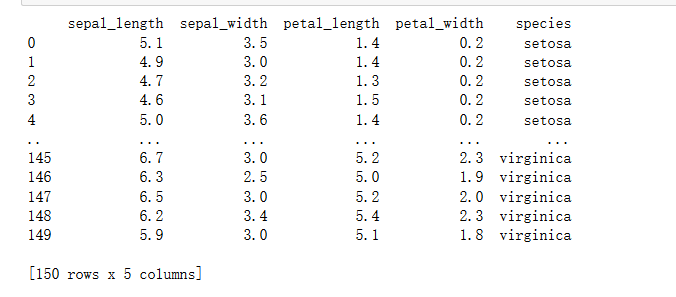### 4、线性回归分析图（与点图相似）

tips = sns.load_dataset("tips")
# can load data on the Internet or from the dist
with sns.axes_style('white'):
sns.regplot(x='total_bill',y='tip',data=tips)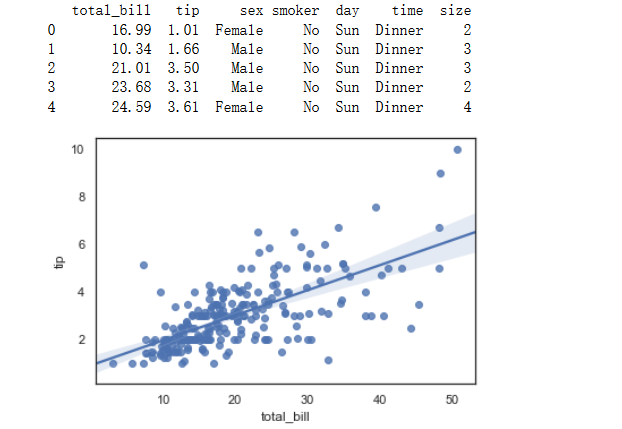regplot相较于implot，没有implot功能丰富，如下例所示：

sns.lmplot(x='total_bill',y='tip',col='day',data=tips,col_wrap=2)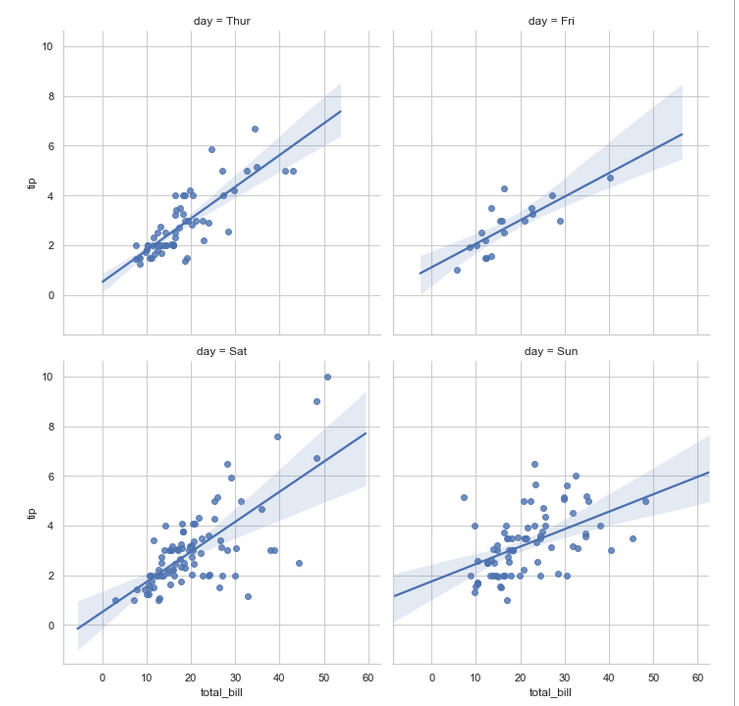### 5、分布散点图

sns.swarmplot(x='day',y='total_bill',data=tips)
sns.swarmplot(x='day',y='total_bill',hue='sex',data=tips)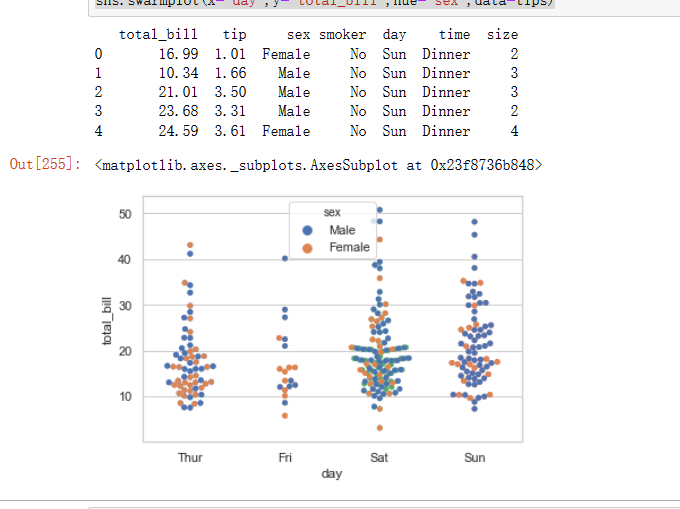### 6、盒图

sns.boxplot(x="day", y="total_bill", hue="time", data=tips);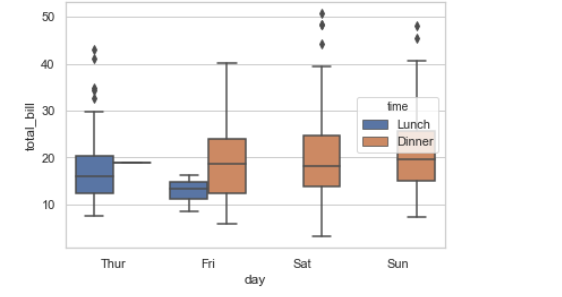### 7、小提琴图

sns.violinplot(x="day", y="total_bill", hue="sex", data=tips, split=True);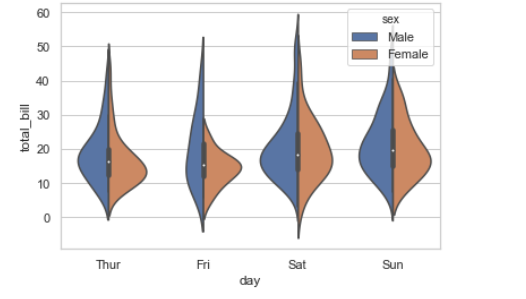sns.violinplot(x="day", y="total_bill", data=tips, inner=None)
sns.swarmplot(x="day", y="total_bill", data=tips, color="w")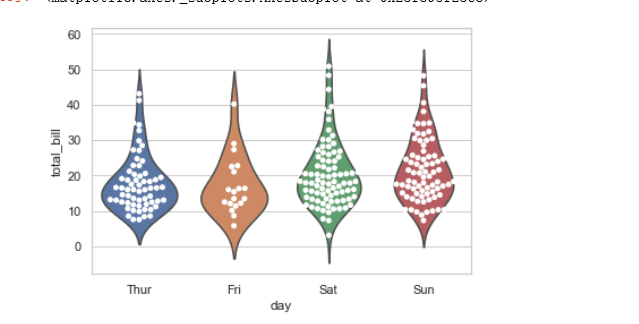### 8、条形图

# 显示值的集中趋势可以用条形图
sns.barplot(x="sex", y="survived", hue="class", data=titanic);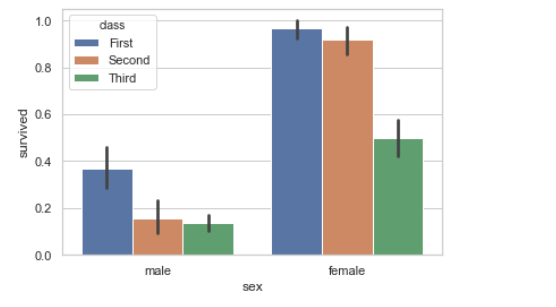### 9、热力图

uniform_data = np.random.rand(3, 3)
print (uniform_data)
heatmap = sns.heatmap(uniform_data)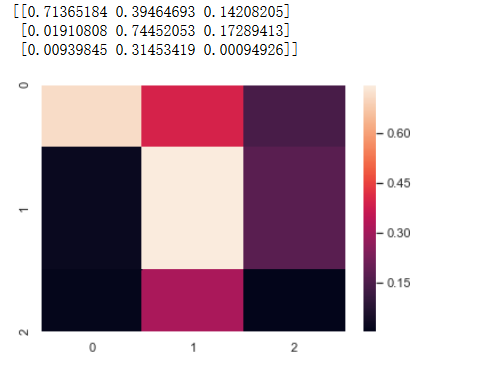flights = sns.load_dataset("flights")
flights = flights.pivot("month", "year", "passengers")
ax = sns.heatmap(flights)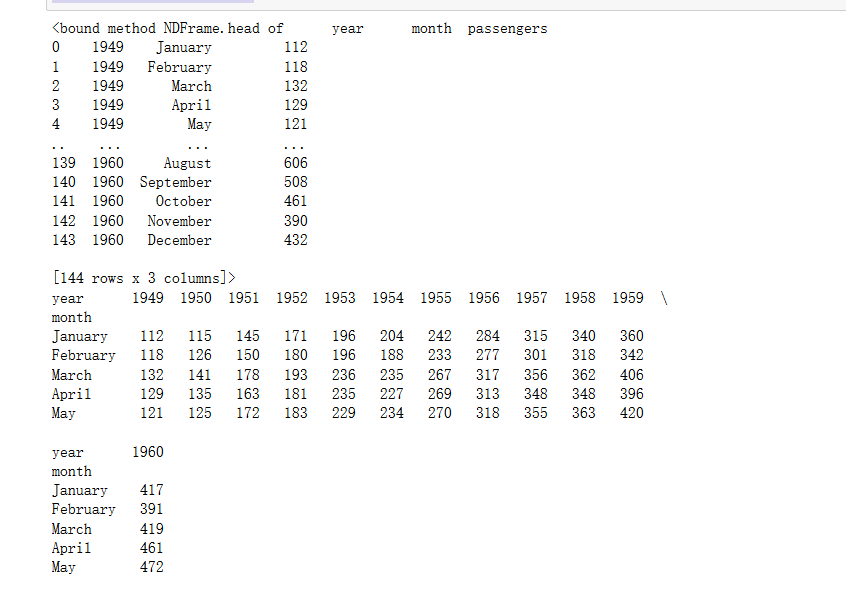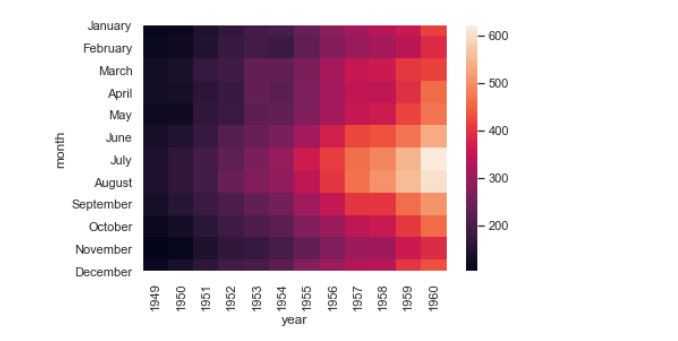ax = sns.heatmap(flights, linewidths=.5)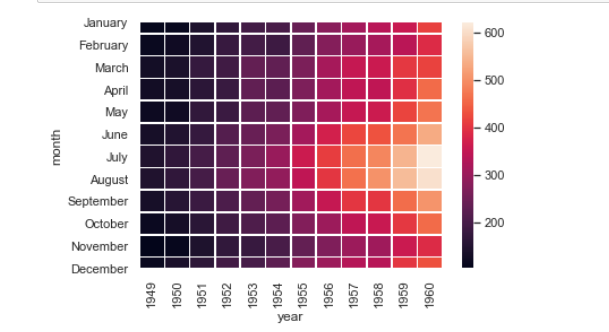## 4、总结

不管是numpy，pandas，还是matlibplot，seaborn数据可视化库，其最终目的只是为了帮助我们进行有效的数据分析。所以比起记各种库的使用，更重要的是我们要掌握数据是如何分析的。对于数据可视化来说，无非就是x，y轴，只要这两个数据具备，就能画图函数得到理想的图像，其余一般只是细节上面的操作，无伤大雅

说起这个，如果我拿到了一组数据可以这样做：

1、通过柱形图或盒图来分析单个变量的密集程度以及内部的关系（一个变量）

2、通过折线图、点图或线性回归分析等等来判断各个变量之间的关系（两个变量）

3、使用stripplot (分布散点图)、violinplot（小提琴图）或特殊柱形图等等来反映三个变量之间的关系，这里一般通过添加           hue=‘dataframe的列名’来实现（三个变量）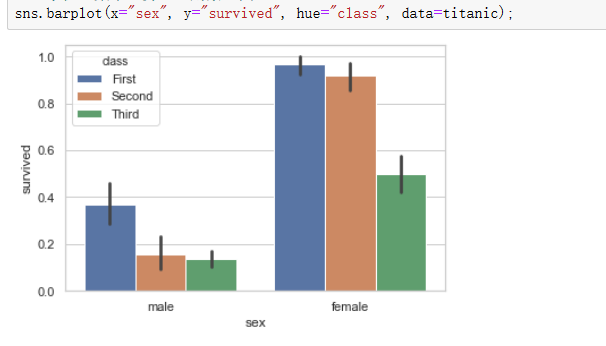4、类似热力图等等很常用的图也值得我们探索。

另：不同的函数画的图反映的只是数据特征，它们均能表示变量之间的关系

如：通过点图和盒图都能反映两变量之间的关系，只是侧重点不一样，点图侧重于反映数据的密集型特征，盒图更能反映数据的离散化特点

#### Python小白也会用的pandas用法总结

08-14

09-244293

#### python之seaborn画图库学习绘制常用的图03-03830

#### searbon使用（绘图函数）

12-237万+

#### Python数据可视化—seaborn简介和实例

02-142万+

#### 使用seaborn绘制漂亮的热度图

04-087845

#### Seaborn库的使用（1）

01-242万+

#### Seaborn常见绘图总结

12-05572

#### python 之seaborn库的基础使用（5种常用图形的使用）

07-211万+

#### Seaborn简单画图(一) -- 散点图

10-124901

#### python中seaborn包常用图形使用

02-14401

#### seaborn画热力图注意的几点问题©️2020 CSDN 皮肤主题: 书香水墨 设计师: CSDN官方博客点击重新获取扫码支付1.余额是钱包充值的虚拟货币，按照1:1的比例进行支付金额的抵扣。
2.余额无法直接购买下载，可以购买VIP、C币套餐、付费专栏及课程。余额充值